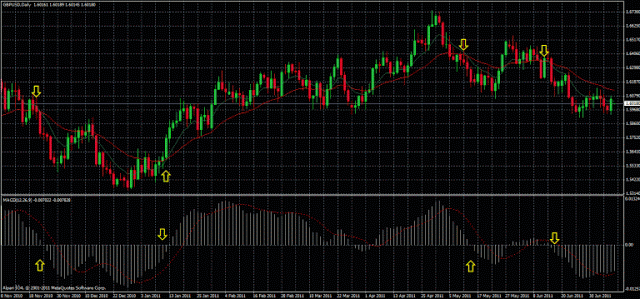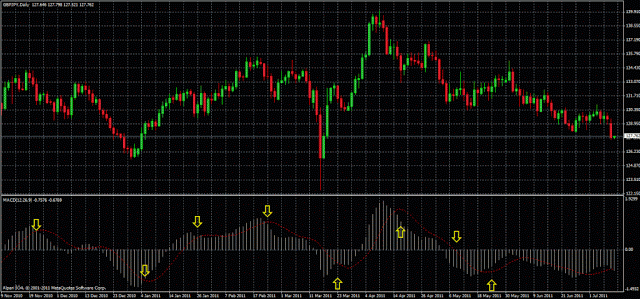# Moving Average Convergence Divergence Indicator – MACD Definition

The Moving Average Convergence Divergence (MACD) is a popular technical indicator used to identify changes in momentum, trend direction, and potential buy/sell signals for an asset. It was developed by Gerald Appel in the late 1970s and has since become a widely used tool by traders and investors.

The MACD is calculated by subtracting a longer-term exponential moving average (EMA) from a shorter-term EMA. The result is a signal line that oscillates around a zero line, which represents the point of equilibrium between buying and selling pressure. The most commonly used EMA periods for the MACD calculation are 12 and 26 days.

In addition to the MACD line, a signal line is typically plotted as a 9-day EMA of the MACD line. This signal line is used to identify potential buy/sell signals based on crossovers with the MACD line. When the MACD line crosses above the signal line, it is considered a bullish signal, suggesting that buying pressure is increasing. Conversely, when the MACD line crosses below the signal line, it is considered a bearish signal, suggesting that selling pressure is increasing.

The MACD can also be used to identify divergences between the indicator and the price chart, which can signal potential trend reversals. For example, if the price is making higher highs but the MACD is making lower highs, it may suggest that the bullish trend is losing momentum and a bearish reversal may be imminent.

Overall, the MACD is a versatile indicator that can be used in a variety of ways to help identify potential buy/sell signals, trend direction, and changes in momentum for an asset. As with any technical indicator, it is important to use the MACD in conjunction with other forms of analysis and risk management strategies to make informed trading decisions.

## Calculation of the MACD and signals

The formula to calculate the MACD is the following:
• MACD = EMA(fast) – EMA(slow)

It is represented as a histogram that is distributed over a central line at 0 and a line called the Signal Line. In the most used configuration, which comes by default on all trading platforms, the fast EMA is of 12 periods, the slow EMA is of 26 periods, and 9 periods for the calculation of the Signal Line.

The value of the histogram is the result of the difference in the value of the fast EMA minus the value of EMA slow, in other words, the value of the divergence of two moving averages. In this way we can deduce the meaning of the MACD histogram:
• MACD above 0: In this case the histogram has positive values, indicating that the fast EMA  is greater than the slow EMA, if we remember the lessons of moving averages, this indicates an uptrend. Let´s suppose a value of 0.0030 for the MACD: this would mean that between fast and slow EMA there is a difference of 30 points, for example, if we have the GBP/USD with a fast EMA of 1.6030 and a slow EMA of 1.6000.
We can also deduce that if the histogram is positive and increasing the uptrend is in progress since the difference between both EMA is widening. Conversely, if the histogram is positive but decreasing, the uptrend may be subsiding as the difference between fast and slow EMA decreases.
• MACD below 0: If the fast EMA is below the slow EMA, the difference between both EMA will be negative and as a result, the MACD will produce values under 0. As you recall from the lessons about moving averages, a fast EMA under a slow EMA indicates a downtrend.
In the same way as before, if the histogram is below 0 and it becomes more negative, that is a sign indicating that the downtrend is in progress since the difference between both EMA is growing.
• MACD Crossing Level 0: If the MACD crosses Level 0 from the bottom up, this is an indication that the fast EMA has changed from being less than the slow EMA to be greater, that is, it is a sign of the crossing of moving averages and the change from downtrend to an uptrend. Similarly, if the MACD crosses the 0 levels from top to bottom indicates the crossing of the fast EMA EMA slowly from top to bottom, this is the reversal of upward to downward.This image shows the points where the MACD crosses the Level 0 and the crosses of the correspondent EMAs

With what has been said you may think: Why do I want the MACD if the intersection of two EMAs and the distance between them can be seen without a new indicator?

For the answer I present the other component of the MACD, the signal line. This line is a simple moving average calculated on the difference of the two EMA, in other words, it is a moving average of the histogram value. As any moving average, it shows the movement of the histogram in a more smoothed way and together with the histogram this is one of the common ways of taking MACD signals: the crossing of the histogram and the signal line. Other signals generated by the MACD and commonly used by the traders is the MACD/Price divergence. Then we will study both types of signals in more detail.

### Crossing of the MACD with the signal line

The signal line, as mentioned before, is a moving average  of 9 ​​periods by default,  on the MACD. The crossing of the signal line with the histogram tell us of a possible reversal in the market trend more rapidly than a crossing of EMAs. In this case we have two possibilities:
• The intersection is generated in the direction in which the histogram is greater than the signal line. This crossing is an indication of a rising market. To catch a more extended market movement is ideal to take this signal when it occurs under the Level 0 .
• The intersection is generated in the direction in which the histogram is smaller than the signal line. This cross gives a possible sell signal. Just as the previous case, to catch a more extended market movement the trader should ideally take this signal when it occurs above of the Level 0.

The following image shows an ideal scenario with the signals of crosses of the MACD with the signal line:### MACD/Price divergence

The divergence between the MACD and the price on the chart is a fairly reliable signal and very commonly used by a lot of traders.

MACD Bullish Divergence: This divergence will give us a buy signal and is produced when there are two minimum in the price,  the second lower than the first, while the two corresponding minimum in the MACD are in ascending order, in other words, the second minimum in the MACD is greater than the first. Although the instrument´s price continues declining, which is visible with minimums that are decreasing, the fact that the minimum for the MACD are increasing indicates that the momentum is approaching to an upward trend.

And since a picture is worth a thousand words, here’s an image with a bullish divergence: